# Euclidean distance, allocation and other stuff...

804
3
10-03-2018 10:48 PM
Labels (1)byMVP Esteemed Contributor
2 3 804

Your standard fare in raster world

Fill in some holes … 'buffer' a line... allocate space to stuff in space... identify unique regions

Fancy names.  Nibble, Euclidean distance, Euclidean allocation, Regiongroup

Start with a raster or array.  Fancy labels in the top left, some random-ish color scheme with values noted in the middle.

Now, zero ( 0 ) we will say is nodata.  The other numbers represent some class value.

Fill in the 'gaps'... aka, nodata, with the value of a cell with data based on the closest distance.  Euclidean, crow flies, for our purposes, but it need not be.

Go!

What did you get for cells A04, H01 and H02?  What about cell D07 and H08?---- (1) The reveal ----

Let's see how we did

A04 - 2   can't be 1 because the diagonal to '1' is 1.414*cell width, so 2 it is

H01 - 2   could have been a 2 or a 3, because both are 3 cells away from cells with values

H02 - 3   no brainer

D07 - 3   could have been 3 or 4, but 3 wins

H08 -3    3 is 2 cells away and 1 and 5 are a greater distance on an angle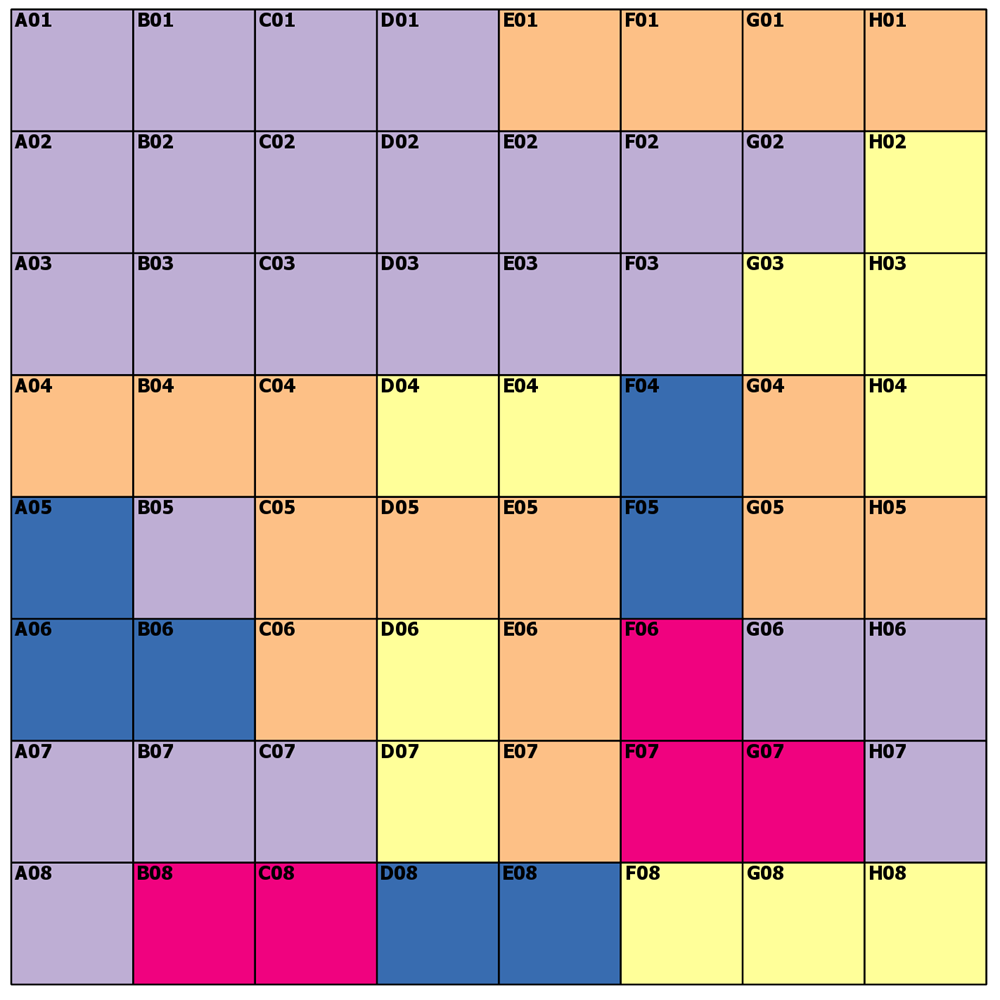---- (3) The distances ----

Pretty boring and obvious... but for completeness.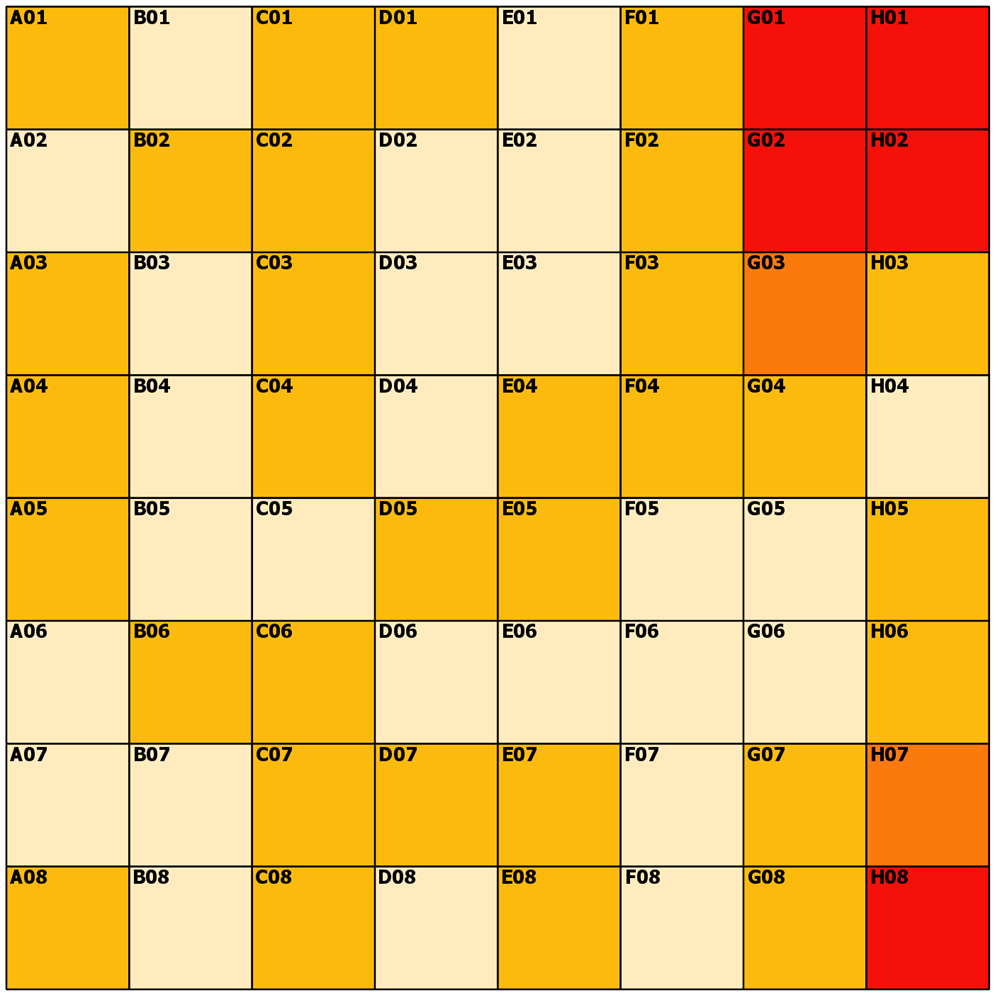I don't do maps, but the dominant colors are 0 or root 2 as you can see from the spiffy ArcGIS Pro symbology tab.So no big surprises---- (4) Allocation around linear features ----

Yes, that is possible too, sort of like a variable buffer, trade area, but currently just a simple Euclidean spatial allocation.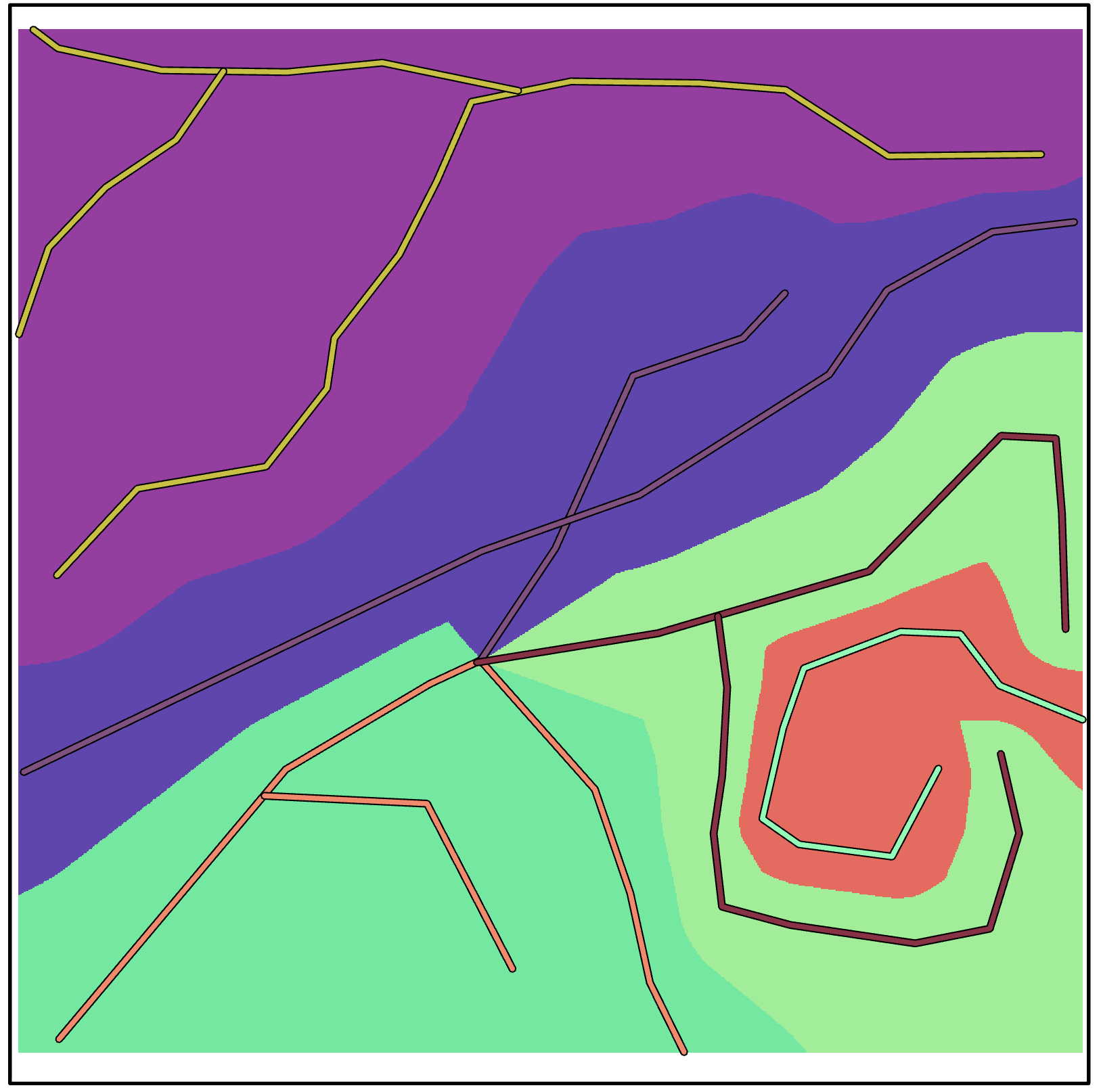---- (5) The references ----

Euclidean Allocation

NumPy/SciPy/  plus Arcpy stuff solution is what I used

``import sysimport numpy as npfrom scipy import ndimage as ndfrom arcpy.geoprocessing import envfrom arcpy.arcobjects import Pointfrom arcgisscripting import NumPyArrayToRaster, RasterToNumPyArrayenv.overwriteOutput = Truedef euclid_calc(a, mask_vals=0, dist=True, alloc=True):    """Calculate the euclidean distance and/or allocation    Parameters:    a : array        numpy float or integer array    mask_vals : number, list or tuple        If a single number is provided, a `mask` will be created using it.  A        list or tuple of values can be used to provide multiple value masking.    dist : boolean        True, the distance of the closest non-masked value to the masked cell    alloc : boolean        True, the value of the closest non-masked value to the masked cell    """    if not dist:        dist = None    if not alloc:        alloc = None    m = np.isin(a, mask_vals).astype('int')    dist, idx = nd.distance_transform_edt(m, return_distances=True,                                          return_indices=True)    alloc = a[tuple(idx)]    return dist, alloc# ----------------------------------------------------------------------# .... final code sectionif len(sys.argv) == 1:    testing = True    r_in = r"C:\GIS\A_Tools_scripts\Raster_tools\Data\poly.tif"    a = RasterToNumPyArray(r_in)    dist, alloc = euclid_calc(a, mask_vals=0, dist=True, alloc=True)else:    testing = False    r_in = sys.argv    r_out = sys.argv    LLx = sys.argv    LLy = sys.argv    cell_sze = sys.argv    a = RasterToNumPyArray(r_in)    dist, alloc = euclid_calc(a, mask_vals=0, dist=True, alloc=True)    #    # specify a, dist or alloc below... this could be a tool choice if needed    #    r0 = NumPyArrayToRaster(a, Point(LLx, LLy), cell_sze)      r0.save(r_out)‍‍‍‍‍‍‍‍‍‍‍‍‍‍‍‍‍‍‍‍‍‍‍‍‍‍‍‍‍‍‍‍‍‍‍‍‍‍‍‍‍‍‍‍‍‍‍‍‍‍‍‍‍‍‍‍‍‍‍‍‍‍‍‍‍‍‍‍‍‍‍‍‍‍‍‍‍‍‍‍‍‍‍‍‍‍‍‍‍‍‍‍‍‍‍‍‍‍‍‍‍‍‍‍‍‍‍‍``

Of course, when run from within the amazing Spyder python IDE, you can simply skip saving to output raster if needed, or try various other options from with the scipy slate other than the distance_transform_edt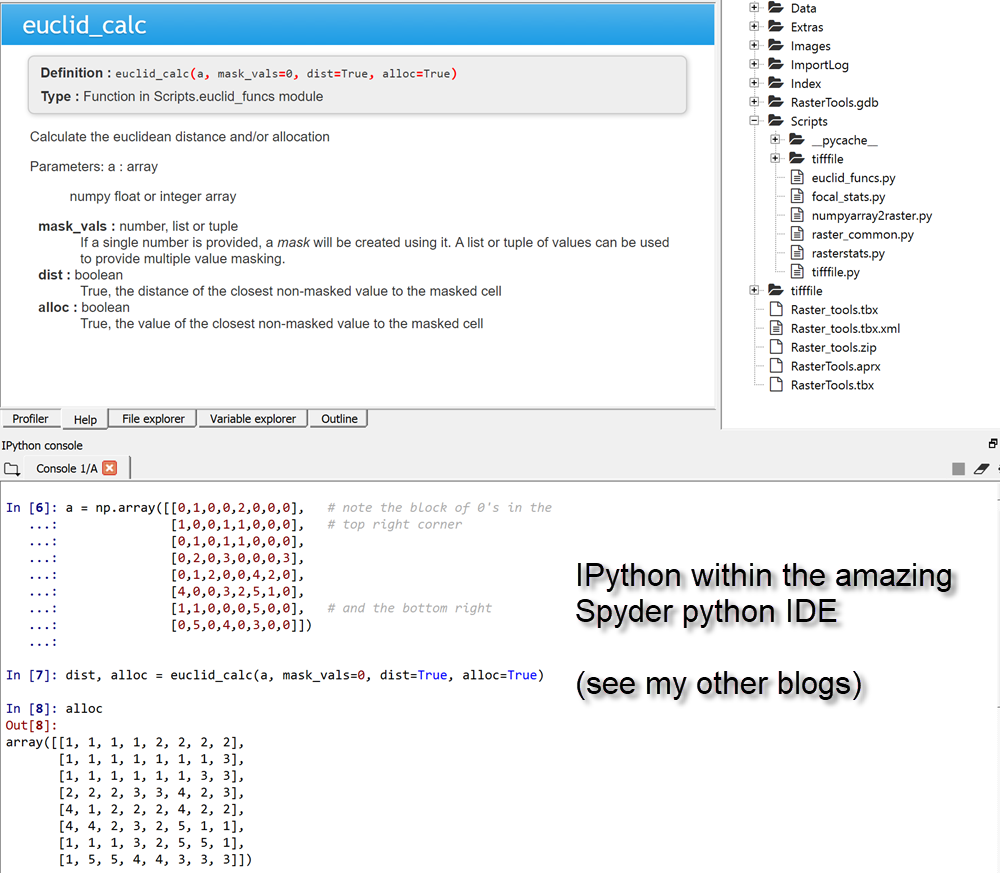On to other "special analyst" tools soon.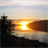New Contributor III

This is quite interesting Dan, I have not written my own Euclidean Allocation but I have my own Euclidean Distance and direction. we will have to chat more as I do a lot of this on a daily basis and would be beneficial to pick each other's brains.

I use a pretty simple method of getting a range of the x and y coordinates and I use numpy broadcasting to get the distance from all the others relative to a source cell. I posted an example of a possible output image. with the source being the little green dot.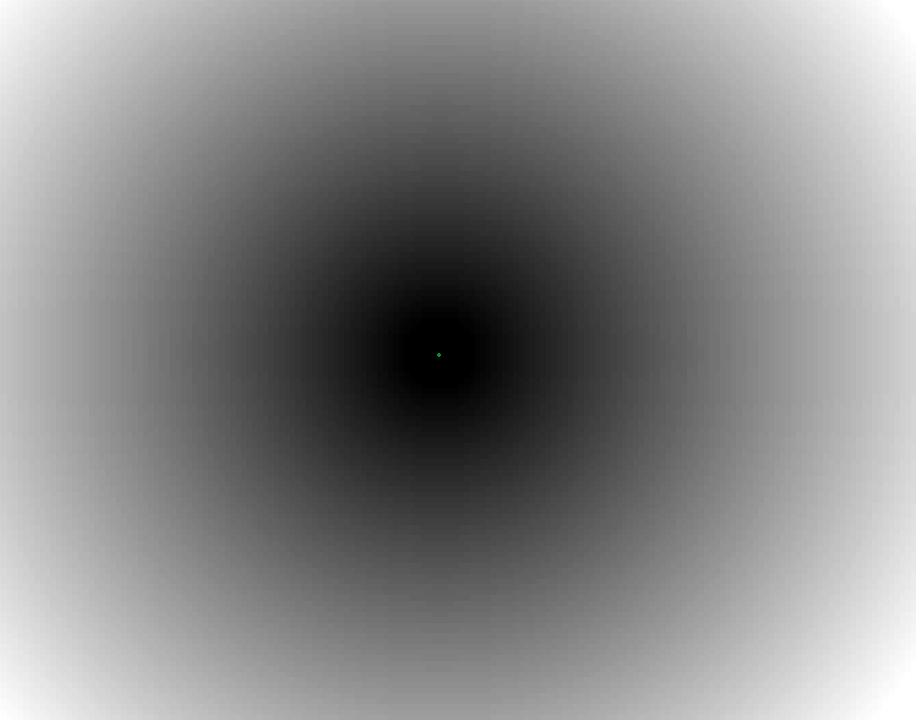byMVP Esteemed Contributor

Nice!  Sometimes I just use meshgrid to emulate cell locations.

``c = np.array([5,5])shp = 11m = np.meshgrid(np.arange(shp), np.arange(shp))xy = np.array(list(zip(m.ravel(), m.ravel())))c = np.array([5,5])e_dist(c, xy).reshape(shp, shp)array([[7.07, 6.4 , 5.83, 5.39, 5.1 , 5.  , 5.1 , 5.39, 5.83, 6.4 , 7.07],       [6.4 , 5.66, 5.  , 4.47, 4.12, 4.  , 4.12, 4.47, 5.  , 5.66, 6.4 ],       [5.83, 5.  , 4.24, 3.61, 3.16, 3.  , 3.16, 3.61, 4.24, 5.  , 5.83],       [5.39, 4.47, 3.61, 2.83, 2.24, 2.  , 2.24, 2.83, 3.61, 4.47, 5.39],       [5.1 , 4.12, 3.16, 2.24, 1.41, 1.  , 1.41, 2.24, 3.16, 4.12, 5.1 ],       [5.  , 4.  , 3.  , 2.  , 1.  , 0.  , 1.  , 2.  , 3.  , 4.  , 5.  ],       [5.1 , 4.12, 3.16, 2.24, 1.41, 1.  , 1.41, 2.24, 3.16, 4.12, 5.1 ],       [5.39, 4.47, 3.61, 2.83, 2.24, 2.  , 2.24, 2.83, 3.61, 4.47, 5.39],       [5.83, 5.  , 4.24, 3.61, 3.16, 3.  , 3.16, 3.61, 4.24, 5.  , 5.83],       [6.4 , 5.66, 5.  , 4.47, 4.12, 4.  , 4.12, 4.47, 5.  , 5.66, 6.4 ],       [7.07, 6.4 , 5.83, 5.39, 5.1 , 5.  , 5.1 , 5.39, 5.83, 6.4 , 7.07]])‍‍‍‍‍‍‍‍‍‍‍‍‍‍‍‍‍‍‍‍‍‍‍``

And a helper function that I have posted before (part of my inprogress, arraytools on GitHub) is below.

import numpy as np  # of course

``def e_dist(a, b, metric='euclidean'):    """Distance calculation for 1D, 2D and 3D points using einsum    `a`, `b` : array like        Inputs, list, tuple, array in 1, 2 or 3D form    `metric` : string        euclidean ('e', 'eu'...), sqeuclidean ('s', 'sq'...),    -----------------------------------------------------------------------    """    a = np.asarray(a)    b = np.atleast_2d(b)    a_dim = a.ndim    b_dim = b.ndim    if a_dim == 1:        a = a.reshape(1, 1, a.shape)    if a_dim >= 2:        a = a.reshape(np.prod(a.shape[:-1]), 1, a.shape[-1])    if b_dim > 2:        b = b.reshape(np.prod(b.shape[:-1]), b.shape[-1])    diff = a - b    dist_arr = np.einsum('ijk,ijk->ij', diff, diff)    if metric[:1] == 'e':        dist_arr = np.sqrt(dist_arr)    dist_arr = np.squeeze(dist_arr)    return dist_arr‍‍‍‍‍‍‍‍‍‍‍‍‍‍‍‍‍‍‍‍‍‍‍‍‍``

at may seem like overkill for small arrays, but I have used it for calculating distance from an origin to multiple destinations in the millions in under a second..

It scales well also, array a and b can be of any shape, so it emulates SciPy's pdist, cdist and the other one...

hence the e_dist (an homage to Einstein and einsum)byMVP Esteemed Contributor

So if you have some pure raster, please a great idea to share and collaborate